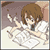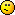Discussion about math, puzzles, games and fun.   Useful symbols: ÷ × ½ √ ∞ ≠ ≤ ≥ ≈ ⇒ ± ∈ Δ θ ∴ ∑ ∫  π  -¹ ² ³ °

You are not logged in.

#1 2014-11-18 20:52:58

azair
MemberRegistered: 2013-01-08
Posts: 5

Reducing the Expression of Time Complexity!!!

Hello Everyone,
I was trying to understand the calculation regarding the Time-Complexity of an Algorithm, but got stuck @line no :04 and 06
Here is the problem :
01:   T(n)=T(n-1)+T(n-2)+4  and T(0)=T(1)=1
02:   Lets assume that T(n-1)~T(n-2)
03:   then, T(n)=2T(n-2)+c   where c=4
04:   2{2T(n-4)+c}+c   <-- HOW???05:   4T(n-4)+3c
06:   2{4T(n-4)+3c}+c  <-- HOW??? and HOW "...3c}+c"???07:   16T(n-8)+15c

and therefore,

08:   T(n)=2^k T(n-2k)+(2^k -1)c
09:   T(n)=2^n/2 T(0) + (2^n/2 -1)c  since: n-2k=0; k=n/2.
10:   T(n)=(1+c)2^k - c.

=>I just don't understand as to what is happening @line 04 and 06.
Can anyone help me?

☁ ♪ ☁☁♪♫☁
♫☁☁♪ ☁
❄ Music in the Sky ❅

Offline

#2 2014-11-18 21:45:18

bob bundyRegistered: 2010-06-20
Posts: 8,523

Re: Reducing the Expression of Time Complexity!!!

hi azair

01:   T(n)=T(n-1)+T(n-2)+4  and T(0)=T(1)=1
02:   Lets assume that T(n-1)~T(n-2)
03:   then, T(n)=2T(n-2)+c   where c=4
There's a line missing here that should make it clearer.
replace n by n-2 in line 03
03.5: T(n-2) = 2T(n-4) + c
Now replace T(n-2) in line 03 using line 03.5

04:   T(n) = 2{2T(n-4)+c}+c
and simplfy
05:   =4T(n-4)+3c
Another missing line here
replace n by n-2 again
05.5: T(n-2) = 4(n-6) + 3c
but, from line 03,
T(n) = 2T(n-2) + c
and replace T(n-2) using line 05.5
And then I think there's a typo; my correction in red

06:  T(n) = 2{4T(n-6)+3c}+c
and simplify
06.5: = 8T(n-6) + 7c
and with n replaced by n-6 in line 03
06.75: T(n-6)=2T(n-8)+c
and replace T(n-6) in line 06.5
06.8: T(n) = 8(2T(n-8) + c) + 7c
and finally simplify to get

07:   T(n) = 16T(n-8)+15c

By now you can, hopefully, spot a pattern in the two numbers:

2,4,8,16,.......powers of 2

and 1,3,7,15,......powers of 2 minus 1

which is where the general formula comes from.

Hope that helps,Bob

Last edited by bob bundy (2014-11-18 21:50:45)

Children are not defined by school ...........The Fonz
You cannot teach a man anything;  you can only help him find it within himself..........Galileo Galilei
Sometimes I deliberately make mistakes, just to test you!  …………….Bob BundyOffline

#3 2014-11-19 01:13:42

azair
MemberRegistered: 2013-01-08
Posts: 5

Re: Reducing the Expression of Time Complexity!!!

Ohhh!! yes!!! I got it...HurrayThank You very much Bob.

☁ ♪ ☁☁♪♫☁
♫☁☁♪ ☁
❄ Music in the Sky ❅

Offline